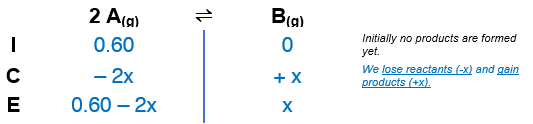# Problem: Consider the reaction of A to form B: 2A(g) ⇌ B(g); Kc = 1.9 × 10−5 (at 298 K)A reaction mixture at 298 K initially contains [A] = 0.60 M. What is the concentration of B when the reaction reaches equilibrium?

###### FREE Expert Solution

We’re given the following equilibrium reaction:

2 A(g) ⇌ B(g); Kc = 1.9 × 10−5 (at 298 K)

From this, we can construct an ICE table:The Kc expression for the reaction is:

$\overline{){{\mathbf{K}}}_{{\mathbf{c}}}{\mathbf{=}}\frac{\mathbf{product}}{\mathbf{reactant}}{\mathbf{=}}\frac{\left[B\right]}{{\left[A\right]}^{\mathbf{2}}}}$

94% (26 ratings)###### Problem Details

Consider the reaction of A to form B: 2A(g) ⇌ B(g); Kc = 1.9 × 10−5 (at 298 K)
A reaction mixture at 298 K initially contains [A] = 0.60 M. What is the concentration of B when the reaction reaches equilibrium?

Frequently Asked Questions

What scientific concept do you need to know in order to solve this problem?

Our tutors have indicated that to solve this problem you will need to apply the ICE Chart concept. You can view video lessons to learn ICE Chart. Or if you need more ICE Chart practice, you can also practice ICE Chart practice problems.

What professor is this problem relevant for?

Based on our data, we think this problem is relevant for Professor Hove's class at RUTGERS.